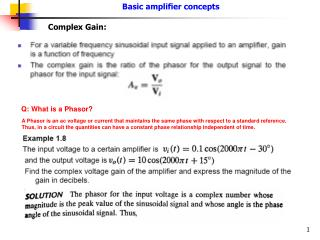DownloadDownload PresentationComplex Gain:

# Complex Gain:

Télécharger la présentation## Complex Gain:

- - - - - - - - - - - - - - - - - - - - - - - - - - - E N D - - - - - - - - - - - - - - - - - - - - - - - - - - -
##### Presentation Transcript

1. Basic amplifier concepts Complex Gain: Q: What is a Phasor? A Phasor is an ac voltage or current that maintains the same phase with respect to a standard reference. Thus, in a circuit the quantities can have a constant phase relationship independent of time.

2. Basic amplifier concepts

3. Basic amplifier concepts │Avmid │ │Avmid │ • AC coupled amplifiers will always have zero gain at dc voltage. Examples include audio amplifiers and ECG amplifiers, where application of dc signal is undesirable. • A DC-coupled amplifier will have a constant gain down to very low frequencies and also dc. Example include amplifiers for video signals.

4. Basic amplifier concepts The gain of an amplifier reduces at higher frequencies. This is due to the parasitic capacitance and inductances as shown in Fig. 1.38. Stray capacitances occur between the conductor and the ground, while stray inductances happen due to the conductor surrounded by a magnetic field (created by current flow).

5. Basic amplifier concepts Voltage gain reducing by 1/2 times indicates that power will be reduced by half, at the half power frequencies.

6. Basic amplifier concepts However, often the signal contain a “common” voltage applied to both terminals that also affects the output signal. This is not desirable, and a good amplifier needs to minimize such effects.

7. Basic amplifier concepts The patient has a common mode input due to the parasitic capacitances. The ECG machine must be able to ignore the common mode signal and not amplify it.

8. Basic amplifier concepts

9. Basic amplifier concepts

10. Basic amplifier concepts 1. Measure the common mode gain Acm 2. Measure the differential gain Ad 3. Compute the CMRR = 20 log ‌Ad/Acm

11. P1.17: An FM radio receiver has an input resistance of 75 Ohm. The input signal provided by a distant transmitter is 5 µV rms, and the receiver supplies 5 V rms to an 8 Ω loudspeaker. Calculate the power gain of the receiver. P1.33: An amplifier has a voltage gain of 30 dB, and a current gain of 70 dB. What is the power gain in dB? If the input resistance is 100 KΩ, what is the load resistance? P1.63: A differential amplifier has vo (t) = A1v1(t) – A2v2(t), If A1 = 1000, and A2 = 999, determine the CMRR. Answers: P1.17: 9.375 x 1E12 P1.33:GdB = 50, RL = 1 KΩ P1.63: CMRR = 60 dB Practice Problems Answer: -20 dB and CMRR = 114 dB